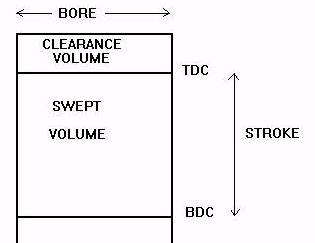## Thanks To David Baker At www.PumaRacing.co.uk For This Information

There are often questions in newsgroups about how to calculate engine capacity and compression ratio. Rather than rely on charts or rough estimates it is very simple to do all the calculations yourself.The piston sweeps up and down inside the cylinder from Top Dead centre (TDC) to Bottom Dead Centre (BDC). The distance it travels vertically doing this is known as the "Stroke" of the engine and this is determined by the length of the crankshaft throw. The volume swept out is known as the "Swept Volume" and this is the capacity of each cylinder. Above the piston at TDC is the volume contained in the cylinder head gasket and combustion chamber - this volume is called the "Clearance Volume". Each time the piston goes through a cycle it compresses all the fuel air mixture sucked into the engine into the clearance volume before ignition takes place.

The volume of a cylinder is calculated by multiplying the area of the bore of the cylinder by the length. In the case of an engine the length is the stroke length. The bore area is calculated as follows:

Bore Area = Bore x Bore x pi / 4. (pi has a value of 3.14159)

Normally we measure engine volume in cubic centimetres although engine dimensions are often shown in millimetres. We need to convert any measurements into centimetres before starting the calculations. Lets take the example of a VW Gti 1800 cc engine. The bore is 81mm and the stroke is 86.4mm. Converting into centimetres we get Bore = 8.1cm, stroke = 8.64cm.

Bore area is therefore 8.1 x 8.1 x 3.14159 / 4 = 51.53 square cm.

Volume of 1 cylinder is bore area times stroke = 51.53 x 8.64 = 445.22 cc

As there are 4 cylinders in the engine the total engine volume is 445.22 x 4 = 1780.88 cc

### COMPRESSION RATIO

The compression ratio is defined as (Swept Volume + Clearance Volume) / Clearance Volume

We already know the swept volume of each cylinder from above - let's assume the clearance volume of each cylinder is 55.6cc - what is the compression ratio?

Compression ratio = (445.22 + 55.6) / 55.6 = 9.0

If we divide the swept volume alone by the clearance volume we get a number which is 1 less than the compression ratio:

445.22 / 55.65 = 8.0

This makes it easy to calculate the clearance volume we need in order to obtain a given compression ratio. What clearance volume do we need if we want to raise the compression ratio of the engine to 10.0? We simply divide the swept volume by 1 less than the compression ratio we need to obtain:

445.22 / 9.0 = 49.5cc for a compression ratio of 10.0. Thus we need to skim the head or fit higher compression pistons until the clearance volume drops from 55.6cc to 49.5cc.